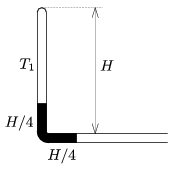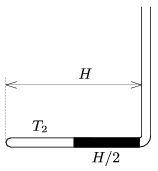Mathematical and Physical Journal
for High Schools
Issued by the MATFUND Foundation
 Already signed up? New to KöMaL?

#Problem P. 4418. (February 2012)

P. 4418. One end of a thin L-shaped tube is sealed, and the tube is held in the vertical plane. The mercury column of length H/2 confines some air (as shown in Figure 1.), the temperature of the confined air is T1=279 K. The external air pressure p0 is equal to the hydrostatic pressure of a mercury column of height H=76 cm. a) Determine the pressure of the confined air.Figure 1

b) The tube is turned by 90o, and the air is cooled down such that the air is in the horizontal arm of the L-shaped tube as shown in Figure 2. What is the temperature T2 of the air now?Figure 2

(4 pont)

Deadline expired on March 12, 2012.

Sorry, the solution is available only in Hungarian. Google translation

Megoldás. $\displaystyle a)$ $\displaystyle p_{1}=0{,}76\cdot10^5~{\rm Pa}=57$ Hgcm.

$\displaystyle b)$ $\displaystyle T_{2}=\frac{8}{9}T_{1}=248$ K.

### Statistics:

 133 students sent a solution. 4 points: 78 students. 3 points: 30 students. 2 points: 14 students. 1 point: 7 students. 0 point: 2 students. Unfair, not evaluated: 2 solutionss.

Problems in Physics of KöMaL, February 2012